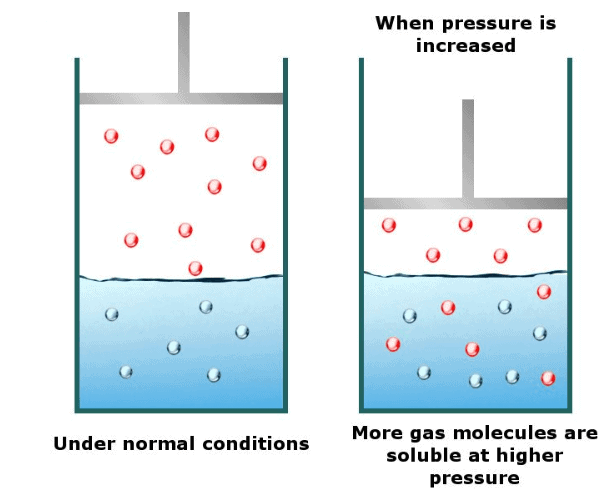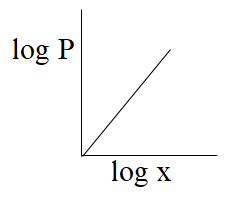# Factors Affecting SolubilityA solution is actually a type of mixture formed when one or more substances dissolve into another. It is a homogeneous mixture as it has a uniform composition throughout.

The substance that dissolves is called solute while the substance in which the solute is dissolved is known as a solvent. There can be one or more types of solutes present in a solution.

The different molecules in a solution cannot be distinguished by naked eyes. The solute particles in the solution are so small in size that they cannot be separated even by using the filtration process.

Also, a solution is a stable entity and the different molecules, although not chemically combined, do not separate with time.

A few examples of solutions are sugar and water solution, salt and water solution, etc.

Contents

## What is Solubility?

Solubility refers to the maximum amount of solute that can be dissolved in one liter of solvent at a given temperature and pressure.

It is the property of a solute that lets it get dissolved in the solvent. A solute can be present in any state of matter viz. solid, liquid, or gas.

Once the maximum solute concentration has been reached, a solution is called saturated and when the solute starts to precipitate out of the solution, it is known as a supersaturated solution.

Here, it is also important to mention the terms soluble, which means that a particular solute is able to dissolve in a particular solvent, and insoluble, which means that the given solute cannot be dissolved in the given solution.

However, it is to be noted that the solubility of a substance varies with the solvent.

For example, noble metals are insoluble in almost any solvent, though, they are soluble in aqua regia.

The terms soluble and insoluble are replaced with miscible and miscible when both the solute and solvent are in the liquid state.

Based on their solubility the solutes are further divided into three categories:

Highly Soluble: When 0.1 gm or more amount of solute gets dissolved in 100 gm solvent.

Sparingly Soluble: When less than 0.1 gm solute gets dissolved in 100 gm solvent.

Insoluble: When the solute does not dissolve in the solvent at all.## Which Factors Affect Solubility?

The solubility of a solute is governed by a number of factors that differ from one solution to another and due to which a solute might be highly soluble in one solvent, sparingly soluble in another while insoluble in some other solvent.

Therefore, we must understand the three main types of solutions:

### 1. Liquids as Solvent

In such solutions, the solubility is determined by the capacity of the two liquids to form a bond with each other.

For example in ethanol in water solution, the solubility depends upon the formation of hydrogen bonds between the molecules of alcohol and water.

The factors that affect the solubility in such solutions are:

Temperature: As we read in the earlier section, solubility for a particular solution is measured at a certain temperature therefore change in temperature also changes the solubility.

Mostly rise in temperature results in increasing solubility.

However, this increase in solubility is for a range of temperatures, like water dissolves most solutes in the temperature range between 20 and 100°C.

Above this temperature range, the solubility does not increase further.

This graph of increasing solubility may get reversed in the case of gases where the gaseous solute might expand with temperature and slowly escape from the solution with the increasing temperature.

Pressure: For the gaseous solutes pressure plays a rather important role.

The solubility of a gaseous solute in a solution increases with the rise in partial pressure of the gas.

For example, in aerated beverages, carbon dioxide is dissolved in the liquid solvent under high pressure due to which frizz appears when you open a bottle of cold drink.

Bond and other binding forces: The intermolecular forces between the molecules of solute and solvent are very vital in the formation of a solution. If you have ever heard, like dissolves like, it is true (mostly).

For example, water is a polar substance due to which the other polar solutes, like alcohol, easily dissolve it.

Also, as discussed above, the formation of hydrogen bonds between water and alcohol molecules further increases the solubility.

### 2. Solid as Solvent

As discussed above, like dissolves like, therefore, it is possible to have a solution having solid solute and a solid solvent.

Also, the solute or a solvent in a solution is identified by the ratio of the substances combined to form a solution, the substance present in the larger ratio is considered a solvent.

For example, brass is an alloy of zinc and copper, as it contains 64% copper and 34% zinc, it can be considered a solution of zinc in copper.

To understand the concept of solubility better we need to understand two more terms i.e. dissolution and crystallization.

When the solute particles dissolve in the solvent, the process is called dissolution.

However, some of the solute particles separate out from the solution due to their collision with the solvent particles this is known as the crystallization process.

After some time a state of dynamic equilibrium is achieved in the solution between the process of dissolution and crystallization which act equal and opposite to each other.

Therefore, the number of solute molecules getting dissolved in the solvent becomes equal to the number of solute molecules separating out from the solution.

At this point the solution is called saturated solution and the concentration of solute present in this solution at that point, at a given temperature and pressure is known as solubility. The factors affecting solubility in such solutions are

Temperature: In such solutions also temperature plays a very important role depending on the nature of the dissolution process.

This means that if the dissolution process is endothermic the solubility increases with the increase in temperature.

However, if the process of dissolution is exothermic the rise in temperature results in the decline in solubility.

This is in accordance with Le Chatelier’s principle which is discussed in the following section.Pressure: In such solutions, the pressure does not have much impact on the solubility as solids are usually incompressible.

### 3. Gas as Solvent

In the case of gaseous solution other than the general factors nature of solute and solvent also determine the solubility.

The gases are usually soluble, however, few gases do not dissolve at all and are unable to form a solution.

For example, hydrogen chloride or ammonia readily forms a solution with water, though oxygen does not easily dissolve in water.

The factors affecting the solubility in such solutions are:

Temperature: Here also the temperature affects the solubility in accordance with Le Chatelier’s principle and equilibrium shifts or adjusts to maintain balance in the process.

Pressure: In the case of gaseous solutions, pressure affects the solubility considerably.

An increase in pressure results in a decrease in the distance between the solute molecules.

This means that more solute molecules can be accommodated in a smaller volume.

This results in a rise in the concentration of solute molecules in a solution, thereby, increasing the solubility.## Laws Affecting the Solubility of a Substance

In the above section, we have learned about the two laws involved in estimating the solubility of a particular solution.

In this section, we will try to learn the basic concepts of these laws.

### • Henry’s Law

According to this law, the extent of pressure applied to a solution is directly proportional to the solubility of the gaseous solute in the liquid solvent.

The equation for this law is given by:

P = KHX

Where, P = partial pressure of the gaseous solute

KH = Henry’s law constant

X = mole fraction of gas in liquid solution### • Le Chatelier’s Principle

It was named after French chemist Henry Louis Le Chatelier.

This is also known as equilibrium law as it is used to study the effect of change in certain factors when a system is in equilibrium.

It states that the equilibrium adjusts itself in a forward or backward direction in an attempt to accept the changes.## How to calculate the solubility of a substance?

As we have discussed before, solubility is basically the amount of solute dissolved in a particular solvent at a given temperature and pressure.

It is either calculated in the amount of solute in grams per 100 gram of solvent i.e. gm/100 gm or in the number of moles of solute per liter of solution.

Here, it is assumed that the temperature and pressure in the given solution are uniform throughout. The formula for solubility is expressed as:

S = √Ksp

Where Ksp is the solubility product constant.

Let us try to understand this through an example,

Suppose we have to calculate the solubility product of barium sulfate for which the value of solubility is 1.05 x 10-5 mol dm-3 at 298 K temperature.

Here, we shall first write the solubility equation for BaSO4:

BaSO4 (aq)   <======>    Ba2+ (aq)   +    SO42-(aq)

From the above equation, we can understand that dissolving 1 mole of BaSO4 results in the formation of 1 mole Ba2+ ion and 1 mole SO42- ion.

This means that, [Ba2+] = 1.05 x 10-5 mol dm-3

[SO42-] = 1.05 x 10-5 mol dm-3

Now, placing the values in the solubility equation:

Ksp = [Ba2+] [SO42-]

= 1.05 x 10-5 mol dm-3 X 1.05 x 10-5 mol dm-3

= 1.10 x 10-10 mol2 dm-6

Watch the video elaborating the calculation of solubility.

## Conclusion

Solubility is the maximum amount of solute that can be dissolved in one liter of solvent at a given temperature and pressure.

The factors that affect solubility are temperature, pressure, and the nature of solute and solvent. However, the extent of the effect may vary from one solution to another solution.

The two laws important for understanding solubility are Henry’s law and Le Chatelier’s Principle.

The formula for the solubility of a solute is given by, S = √Ksp, where Ksp is the solubility product constant.